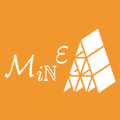### Mathematics in Engineering

2020, Issue 1: 55-74. doi: 10.3934/mine.2020004
Research article Special Issues

# Histogram tomography

• Received: 29 September 2019 Accepted: 22 October 2019 Published: 04 November 2019
• In many tomographic imaging problems the data consist of integrals along lines or curves. Increasingly we encounter "rich tomography" problems where the quantity imaged is higher dimensional than a scalar per voxel, including vectors tensors and functions. The data can also be higher dimensional and in many cases consists of a one or two dimensional spectrum for each ray. In many such cases the data contain not just integrals along rays but the distribution of values along the ray. If this is discretized into bins we can think of this as a histogram. In this paper we introduce the concept of "histogram tomography". For scalar problems with histogram data this holds the possibility of reconstruction with fewer rays. In vector and tensor problems it holds the promise of reconstruction of images that are in the null space of related integral transforms. For scalar histogram tomography problems we show how bins in the histogram correspond to reconstructing level sets of function, while moments of the distribution are the x-ray transform of powers of the unknown function. In the vector case we suggest a reconstruction procedure for potential components of the field. We demonstrate how the histogram longitudinal ray transform data can be extracted from Bragg edge neutron spectral data and hence, using moments, a non-linear system of partial differential equations derived for the strain tensor. In x-ray diffraction tomography of strain the transverse ray transform can be deduced from the diffraction pattern the full histogram transverse ray transform cannot. We give an explicit example of distributions of strain along a line that produce the same diffraction pattern, and characterize the null space of the relevant transform.

Citation: William R. B. Lionheart. Histogram tomography[J]. Mathematics in Engineering, 2020, 2(1): 55-74. doi: 10.3934/mine.2020004

### Related Papers:

• In many tomographic imaging problems the data consist of integrals along lines or curves. Increasingly we encounter "rich tomography" problems where the quantity imaged is higher dimensional than a scalar per voxel, including vectors tensors and functions. The data can also be higher dimensional and in many cases consists of a one or two dimensional spectrum for each ray. In many such cases the data contain not just integrals along rays but the distribution of values along the ray. If this is discretized into bins we can think of this as a histogram. In this paper we introduce the concept of "histogram tomography". For scalar problems with histogram data this holds the possibility of reconstruction with fewer rays. In vector and tensor problems it holds the promise of reconstruction of images that are in the null space of related integral transforms. For scalar histogram tomography problems we show how bins in the histogram correspond to reconstructing level sets of function, while moments of the distribution are the x-ray transform of powers of the unknown function. In the vector case we suggest a reconstruction procedure for potential components of the field. We demonstrate how the histogram longitudinal ray transform data can be extracted from Bragg edge neutron spectral data and hence, using moments, a non-linear system of partial differential equations derived for the strain tensor. In x-ray diffraction tomography of strain the transverse ray transform can be deduced from the diffraction pattern the full histogram transverse ray transform cannot. We give an explicit example of distributions of strain along a line that produce the same diffraction pattern, and characterize the null space of the relevant transform.###### 通讯作者: 陈斌, bchen63@163.com
• 1.

沈阳化工大学材料科学与工程学院 沈阳 110142## Metrics

Article views(814) PDF downloads(404) Cited by(3)

Article outline

## Other Articles By Authors

• On This Site
• On Google Scholar/DownLoad:  Full-Size Img  PowerPoint